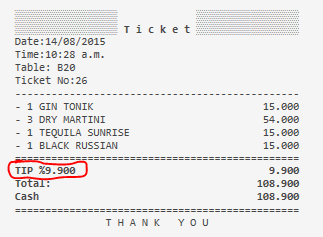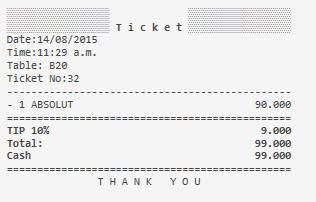# TIP percentage issue in Ticket template

Hi team,

When Im printing an invoice, the TIP detail is showing then of the percentage symbol, the amount of the calculation total, not the real percentage… In this case just something like %10 or 10%…

Is possible remove the detail info then of the % symbol or put the real percentage

ThanksHi Kendash, how r u?

Im using the default ticket template (ticketTemplate.zip (578 Bytes))

So I setup the tips using this forum step by step “How to add tips to ticket”
(How to add tips to ticket)

No need for a ZIP file.
Copy your Template then paste it directly into the forum.
Highlight the text of the Template, then click the `</>` button to turn it into code.

Thanks QMcKay

Im using this default template

``````[LAYOUT]
-- General layout
<T>Ticket
<L00>Date:{TICKET DATE}
<L00>Time:{TIME}
{ENTITIES}
<L00>Ticket No:{TICKET NO}
<F>-
{ORDERS}
<F>=
<EB>
{DISCOUNTS}
{SERVICES}
<J10>Total:|{TICKET TOTAL}
{PAYMENTS}
<DB>
<F>=
<C10>T H A N K   Y O U

[DISCOUNTS]
<J00>{CALCULATION NAME} %{CALCULATION AMOUNT}|{CALCULATION TOTAL}

[SERVICES]
<J00>{CALCULATION NAME}|{CALCULATION TOTAL}

[PAYMENTS]
<J00>{PAYMENT NAME}|{PAYMENT AMOUNT}

[ORDERS]
-- Default format for orders
<J00>- {QUANTITY} {NAME}|{TOTAL PRICE}
{ORDER TAGS}

{ORDER TAGS}

[ORDERS:Void]
-- Nothing will print for void lines

[ORDER TAGS]
-- Format for order tags
<J00> * {ORDER TAG NAME} | {ORDER TAG PRICE}

[ENTITIES:Table]
-- Table entity format
<L00>Table: {ENTITY NAME}

[ENTITIES:Customer]
-- Customer entity format
<J00>Customer: {ENTITY NAME} | {ENTITY DATA:Phone}``````

9.900% of those orders total comes out to 9.801 it looks like your rounding so it rounded to 9.900

So in fact it is working correctly.

EDIT: Nevermind I see what you did.

If you know its 10% then put this.

``````[Discounts]
<J00>{CALCULATION NAME} 10%|{CALCULATION TOTAL:TIP}
``````

Or rename the calculation to TIP 10% and just use:

``````[Discounts]
<J00>{CALCULATION NAME}|{CALCULATION TOTAL:TIP 10%}``````
2 Likes

Hi Kendash

Works so good, thank you!One last question… is possible add 2 default fast Tip buttons like (5%, 10%), that calculates automatically the exact amount?

Yes just instead of using 0 for in the calculation set the exact % you want and then use Calculation Selector for it.

1 Like